# Recall math facts

For each new math fact that we calculate, we get at least one more math fact for free:Gives usand.

Similarly, we can calculate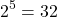This gives us the related factIn this section, it is useful to recall common exponent facts in order to evaluate roots. Consider completing this Reference Sheet for Exponents. Refer to this sheet rather than a calculator for common values.

# Roots and Exponents

Let’s compare what we know about roots and exponents. We know the exponent law that says. We also know thatWe can write that:.

Note that:andIn other words,andare the same number. You can type them both on your calculator and get the same answer.

Extending the idea, we haveIn other words,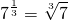And in general:# What about a number that has a power and a root?

Consider a number such asNote that this can also be written as.Therefore,Now, as an exponent, the cube root is the same as the exponent, the first part reads: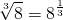The second part is, ‘raise to the power 4’:The an exponent law that reminds us what do to here:. So we can write:When it comes to converting from root notation to exponent notation or vice versa:In general, as long as the base a is a positive number: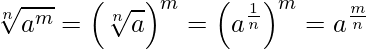# Notation

The idea of powers and roots has been around for a very long time, but the way we write them down and communicate them has changed over the years. You can see from this table, called ‘the origins of some mathematical symbols‘ that the square root symbol,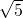has been around since 1524; and exponents as we now use them showed up 100 years later, they have been around since 1637.

We now use both notations interchangeably, but one can note a preference for root notation in geometry and for exponent notation in calculus.

# Evaluating fraction exponents

Generally, a fraction exponent leads to an irrational number. The answers to these examples are integers or rational numbers, and can be evaluated without using a calculator.

Example1:What is the second (square) root of 16?Example 2a:What is the fourth root of 16?For the fourth root you can square root then square root again:Example 2bFirst, we need to know that.

This is how we handle it:

We know that.

And we know thatThis is why we can rewriteasIn this form, we first evaluate the bracket from recall or the reference sheet then calculate the power:Alternatively, convert to root notation then compute:Example 3:We mean, take the cube root of 125 then square it.Example 4We mean, take the square root of 64 then cube.Example 5:The reference sheet shows that. Multiplying by 4 one more time gives..# Evaluate negative exponents

Division can be expressed usingor by using a fraction or by using a negative exponent. A negative exponent does not make the value negative because dividing does not make a value negative.

1 divided by 7 can be written as:Note that all of these are positive (all are equal to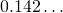). Generally the first thing we do with a negative exponent (which means ‘divide’) is to write it as a fraction.

Example 6:A negative exponent represents repeated division.Example 7:# Fractions with Negative Exponents

In examples 8, 9 and 10 we take a negative power of a fraction. To understand the process, you need to remember how to divide a fraction. Here’s a reminder: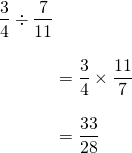Example 8:Watch what happens to the fraction when there is a negative power.Notice that our original fraction flips when the exponent is negative. Let’s write that down in general:

In general,Example 9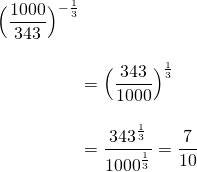Example 10